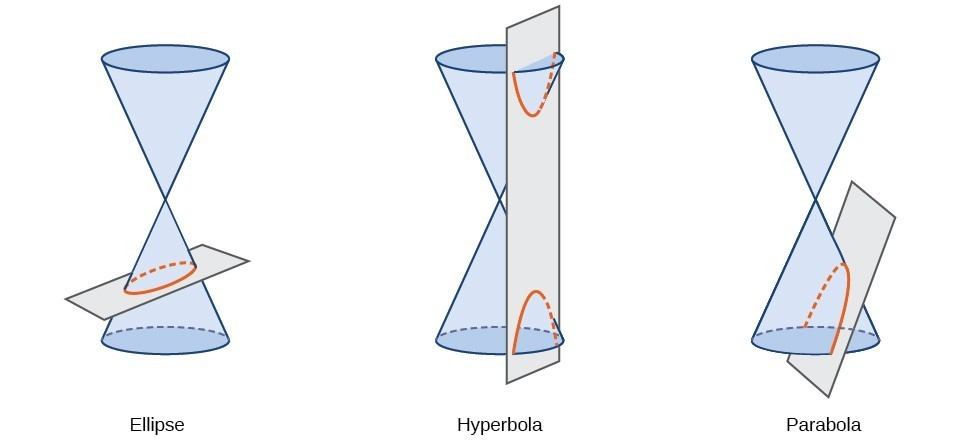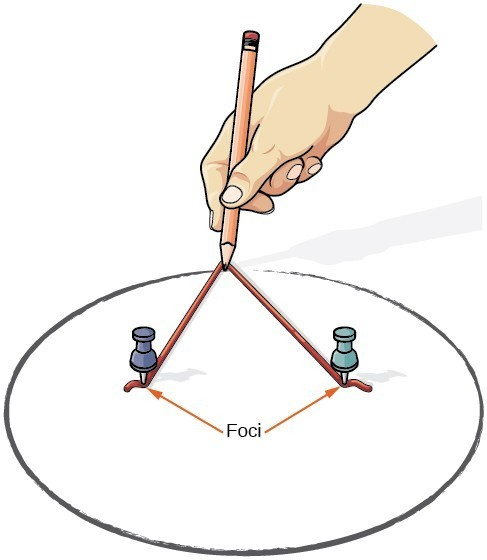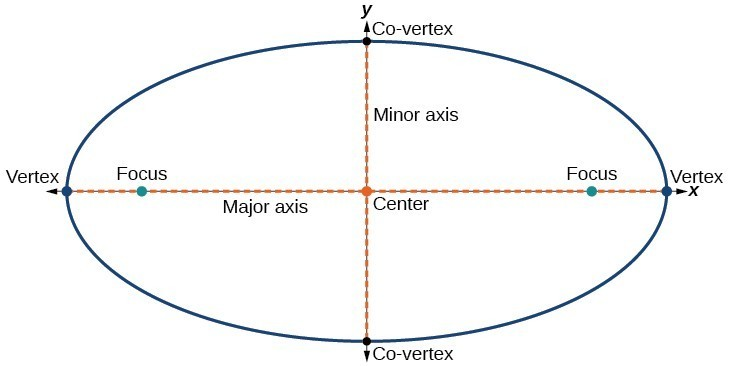## Writing Equations of Ellipses in Standard Form

A conic section, or conic, is a shape resulting from intersecting a right circular cone with a plane. The angle at which the plane intersects the cone determines the shape.Figure 2

Conic sections can also be described by a set of points in the coordinate plane. Later in this chapter, we will see that the graph of any quadratic equation in two variables is a conic section. The signs of the equations and the coefficients of the variable terms determine the shape. This section focuses on the four variations of the standard form of the equation for the ellipse. An ellipse is the set of all points $\left(x,y\right)$ in a plane such that the sum of their distances from two fixed points is a constant. Each fixed point is called a focus (plural: foci).

We can draw an ellipse using a piece of cardboard, two thumbtacks, a pencil, and string. Place the thumbtacks in the cardboard to form the foci of the ellipse. Cut a piece of string longer than the distance between the two thumbtacks (the length of the string represents the constant in the definition). Tack each end of the string to the cardboard, and trace a curve with a pencil held taut against the string. The result is an ellipse.Figure 3

Every ellipse has two axes of symmetry. The longer axis is called the major axis, and the shorter axis is called the minor axis. Each endpoint of the major axis is the vertex of the ellipse (plural: vertices), and each endpoint of the minor axis is a co-vertex of the ellipse. The center of an ellipse is the midpoint of both the major and minor axes. The axes are perpendicular at the center. The foci always lie on the major axis, and the sum of the distances from the foci to any point on the ellipse (the constant sum) is greater than the distance between the foci.Figure 4

In this section, we restrict ellipses to those that are positioned vertically or horizontally in the coordinate plane. That is, the axes will either lie on or be parallel to the x– and y-axes. Later in the chapter, we will see ellipses that are rotated in the coordinate plane.

To work with horizontal and vertical ellipses in the coordinate plane, we consider two cases: those that are centered at the origin and those that are centered at a point other than the origin. First we will learn to derive the equations of ellipses, and then we will learn how to write the equations of ellipses in standard form. Later we will use what we learn to draw the graphs.